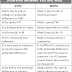The main difference between Volt and Watt is Volt is the SI unit of electrical potential difference or voltage or EMF whereas Watt is the SI unit of electrical power. Current, voltage, and power are three main important parameters for measuring and analyzing electrical energy. Voltage and Current are two parameters among them. Current is the flow of electrons through a conductor whereas voltage is the potential difference or pressure across that conductor that forces to flow the electrons in the form of an electric current.

In different situations, we need to measure different parameters of an electrical system. For example, we need to measure the voltage to know how much dangerous that circuit is and how much insulation we need. On the other hand, we need to measure the current flow to ensure the proper selection of conductor size. Measurement of electrical power helps us to know how much electrical energy is consumed or how much electricity a load can consume.

Here, the key differences between Volts and Watts are given below,

 Volts Watts Volt is the SI unit of voltage or potential difference or EMF Watt is the SI unit of electrical power Volts symbol ‘V’ Watts symbol ‘W’ It is independent of watts Watts dependent on Volts Volt formula, V=IR Watt formula, W= VI Volt is measured by a voltmeter Watt is measured by a wattmeter It is very easy to measure as it just measures the potential difference. It is difficult to measure as both potential difference and current flow need to measure. For the AC system power factor also need to be measured. Volts define the potential difference or electric pressure across a conductor. Watts defines the rate at which electrical energy is transferred from the power source to the load.

Volt rating of any electrical equipment or device helps us to know where we can connect that device. For example, if a device is rated as 230V then we can connect it to a single-phase system. But if the device is rated as 440V then we need to connect it to a three-phase system or a double-phase system. Of course, AC and DC voltage are different. We can't connect a device with a DC power supply that is designed to operate with an AC supply and Vice Versa. Sometimes you may be seen that most of the devices are not rated with a single voltage rating. They have a voltage range such as 120V-230V. It implies that we can connect that device to a 120V power system(used in USA) or a 230V power system(used in India).

The Watt rating of any electrical device or equipment helps us to know how much electrical power will be consumed by that device. For example, if a device is rated as 50 watts, it implies that it will consume 50 watts of electrical power per hour at the rate of 50 joules per second. You may also notice most of the devices have only voltage and power ratings. But from the voltage and power rating, we can calculate how much current it will draw. In the case of a DC device, it is very easy, You just need to divide the wattage by the voltage rating. For example, if a device has a rating of 200 Volts and 600 Watts, then the device will consume the current 600W/200V = 3 amperes. But when you calculate an AC device you must take the power factor into the account.

So, the Volts and Watts ratings are not only helpful to analyze an electrical load but even they are very important for analyzing any electrical system and measurement. With the help of an electrical power rating, we can measure electrical energy consumption. As per the Board of Trade Unit(BoT) 1 unit = 1000 watts X 1 Hour.

Volts VS Watts | The Main DifferencesReviewed by Author on October 24, 2022 Rating: 5